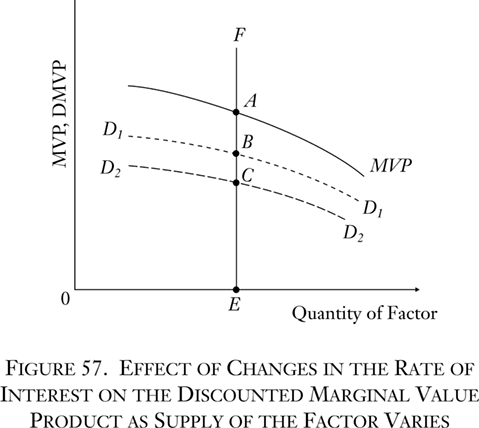# Books / Digital Text

## A. Discounting

If the DMVP schedules determine the prices of nonspecific factor services, what determines the shape and position of the DMVP schedules? In the first place, by definition it is clear that the DMVP schedule is the MVP schedule for that factor discounted. There is no mystery about the discounting; as we have stated, the MVP of the factor is discounted in accordance with the going pure rate of interest on the market. The relation of the MVP schedule and the DMVP schedule may be diagrammed as in Figure 57.The supply of the factor is the EF line at the given quantity 0E. The solid line is the MVP schedule at various supplies. The MVP of the supply 0E is EA. Now the broken line D1D1 is the discounted marginal value product schedule at a certain rate of interest. Since it is discounted, it is uniformly lower than the MVP curve. In absolute terms, it is relatively lower at the left of the diagram, because an equal percentage drop implies a greater absolute drop where the amount is greater. The DMVP for supply 0E equals EB. EB will be the price of the factor in the evenly rotating economy.

Now suppose that the rate of interest in the economy rises, as a result, of course, of rises in time-preference schedules. This means that the rate of discount for every hypothetical MVP will be greater, and the absolute levels lower. The new DMVP schedule is depicted as the dotted line D2D2. The new price for the same supply of the factor is EC, a lower price than before.

One of the determinants of the DMVP schedule, then, is the rate of discount, and we have seen above that the rate of discount is determined by individual time preferences. The higher the rate of discount, the lower will tend to be the DMVP and, therefore, the lower the price of the factor; the lower the interest rate, the higher the DMVP and the price of the factor.